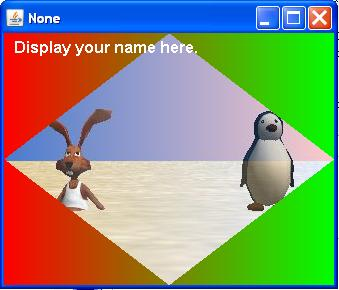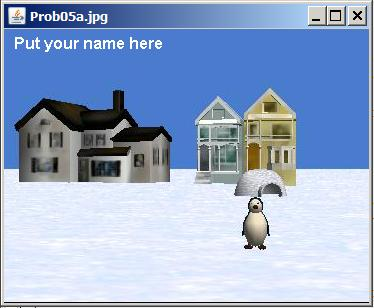# 1.10 Itse1359-1050r-review

 Page 1 / 1
This module contains review questions and answers keyed to the module titled Itse1359-1050-Introduction to Scripts.

## Preface

This module is one in a collection of modules on Python designed for teaching ITSE 1359 Introduction to Scripting Languages: Python at Austin Community College in Austin, TX.

This module contains review questions and answers keyed to the module titled Itse1359-1050-Introduction to Scripts .

Once you study that module, you should be able to answer the review questions in this module.

The questions and the answers in this module are connected by hyperlinks to make it easy for you to navigate from the question to the answer and back again.

(Note to blind and visually impaired students: with the exception of two bitmap images that are used solely as spacers to separate the question sectionfrom the answer section, all of the material in this module is presented in plain text format and should be accessible using an audio screen reader or abraille display.)

## Question 1

In your own words, what is a script?

Go to answer 1

## Question 2

True or False? A script can be used only once and must then be discarded.

Go to answer 2

## Question 3

Convert the interactive program shown in Figure 1 into a script, execute it, and confirm that you get the correct result.

In case you didn't get any output, note that unlike in interactive mode, in order to cause a script to produce an output, you will need to use a statementsomething like the following:

print(x+y)

Figure 1 . Question 3.
```>>>x=6 # create and populate x>>>y=5 # create and populate y>>>x+y # add x to y and display the sum 11>>>```

Go to answer 3

## Question 4

Convert the interactive program shown in Figure 3 into a script and execute it.

Figure 3 . Question 4.
```>>>a=b=c=10 # assign 10 to several variables>>>a+b+c # add them together 30>>>a=b=c=20 # assign 20 to same variables>>>a+b+c # add them together 60>>>```

Go to answer 4

## Question 5

Convert the interactive program shown in Figure 5 into a script and execute it.

Figure 5 . Question 5.
```>>>x=5>>>y=6>>>x+y 11>>>x=5.55555>>>y=6.66666>>>x+y 12.22221>>>```

Go to answer 5

## Question 6

Convert the interactive program shown in Figure 7 into a script and execute it.

Figure 7 . Question 6.
```>>>5+6 11>>>_+22 # add 22 to the continuation variable 33>>>```

Go to answer 6

## Question 7

Convert the interactive program shown in Figure 9 into a script and execute it.

Figure 9 . Question 7.
```>>>print("Dick\nBaldwin") DickBaldwin>>>```

Go to answer 7

## Figure index

What is the meaning of the following two images?

These images were inserted here simply to insert some space between the questions and the answers to keep them from being visible on the screen at thesame time.This image was also inserted for the purpose of inserting space between the questions and the answers.## Answer 7

One possible solution is provided in Figure 10 .

Figure 10 . Answer 7.
`print("Dick\nBaldwin")`

Go back to Question 7

## Answer 6

One possible solution is provided in Figure 8 .

Figure 8 . Answer 6.
```x = 5+6 print(x)x = x + 22 print(x)```

If you were unable to get this to work, don't be dismayed. The special variable whose name is the underscore character is available only ininteractive mode. Therefore, you can't use that variable in a script, and you will need to develop a workaround.

Go back to Question 6

## Answer 5

One possible solution is provided in Figure 6 .

Figure 6 . Answer 5
```x=5 y=6print(x+y) x=5.55555y=6.66666 print(x+y)```

Go back to Question 5

## Answer 4

One possible solution is provided in Figure 4 .

Figure 4 . Answer 4.
```a=b=c=10 # assign 10 to several variables print(a+b+c) # add them togethera=b=c=20 # assign 20 to same variables print(a+b+c) # add them together```

Go back to Question 4

## Answer 3

One possible solution is provided in Figure 2 .

Figure 2 . Answer 3.
```x=6 # create and populate x y=5 # create and populate yprint(x+y) # add x to y and display the sum```

Go back to Question 3

## Answer 2

False. Script files are reusable.

Go back to Question 2

## Answer 1

A script is a text file containing the programming statements that comprise a Python program.

Go back to Question 1

## Miscellaneous

This section contains a variety of miscellaneous information.

Housekeeping material
• Module name: Itse1359-1050r-Review
• File: Itse1359-1050r.htm
• Published: 10/14/14
• Revised: 02/21/15
Disclaimers:

Financial : Although the Connexions site makes it possible for you to download aPDF file for this module at no charge, and also makes it possible for you to purchase a pre-printed version of the PDF file, youshould be aware that some of the HTML elements in this module may not translate well into PDF.

I also want you to know that, I receive no financial compensation from the Connexions website even if you purchase the PDF version ofthe module.

In the past, unknown individuals have copied my modules from cnx.org, converted them to Kindle books, and placed them for sale onAmazon.com showing me as the author. I neither receive compensation for those sales nor do I know who does receive compensation. If youpurchase such a book, please be aware that it is a copy of a module that is freely available on cnx.org and that it was made andpublished without my prior knowledge.

Affiliation : I am a professor of Computer Information Technology at Austin Community College in Austin, TX.

-end-

#### Questions & Answers

what is math number
Tric Reply
x-2y+3z=-3 2x-y+z=7 -x+3y-z=6
Sidiki Reply
Need help solving this problem (2/7)^-2
Simone Reply
x+2y-z=7
Sidiki
what is the coefficient of -4×
Mehri Reply
-1
Shedrak
the operation * is x * y =x + y/ 1+(x × y) show if the operation is commutative if x × y is not equal to -1
Alfred Reply
An investment account was opened with an initial deposit of \$9,600 and earns 7.4% interest, compounded continuously. How much will the account be worth after 15 years?
Kala Reply
lim x to infinity e^1-e^-1/log(1+x)
given eccentricity and a point find the equiation
Moses Reply
12, 17, 22.... 25th term
Alexandra Reply
12, 17, 22.... 25th term
Akash
College algebra is really hard?
Shirleen Reply
Absolutely, for me. My problems with math started in First grade...involving a nun Sister Anastasia, bad vision, talking & getting expelled from Catholic school. When it comes to math I just can't focus and all I can hear is our family silverware banging and clanging on the pink Formica table.
Carole
I'm 13 and I understand it great
AJ
I am 1 year old but I can do it! 1+1=2 proof very hard for me though.
Atone
hi
Adu
Not really they are just easy concepts which can be understood if you have great basics. I am 14 I understood them easily.
Vedant
find the 15th term of the geometric sequince whose first is 18 and last term of 387
Jerwin Reply
I know this work
salma
The given of f(x=x-2. then what is the value of this f(3) 5f(x+1)
virgelyn Reply
hmm well what is the answer
Abhi
If f(x) = x-2 then, f(3) when 5f(x+1) 5((3-2)+1) 5(1+1) 5(2) 10
Augustine
how do they get the third part x = (32)5/4
kinnecy Reply
make 5/4 into a mixed number, make that a decimal, and then multiply 32 by the decimal 5/4 turns out to be
AJ
how
Sheref
can someone help me with some logarithmic and exponential equations.
Jeffrey Reply
sure. what is your question?
ninjadapaul
20/(×-6^2)
Salomon
okay, so you have 6 raised to the power of 2. what is that part of your answer
ninjadapaul
I don't understand what the A with approx sign and the boxed x mean
ninjadapaul
it think it's written 20/(X-6)^2 so it's 20 divided by X-6 squared
Salomon
I'm not sure why it wrote it the other way
Salomon
I got X =-6
Salomon
ok. so take the square root of both sides, now you have plus or minus the square root of 20= x-6
ninjadapaul
oops. ignore that.
ninjadapaul
so you not have an equal sign anywhere in the original equation?
ninjadapaul
hmm
Abhi
is it a question of log
Abhi
🤔.
Abhi
I rally confuse this number And equations too I need exactly help
salma
But this is not salma it's Faiza live in lousvile Ky I garbage this so I am going collage with JCTC that the of the collage thank you my friends
salma
Commplementary angles
Idrissa Reply
hello
Sherica
im all ears I need to learn
Sherica
right! what he said ⤴⤴⤴
Tamia
hii
Uday
hi
salma
hi
Ayuba
Hello
opoku
hi
Ali
greetings from Iran
Ali
salut. from Algeria
Bach
hi
Nharnhar
A soccer field is a rectangle 130 meters wide and 110 meters long. The coach asks players to run from one corner to the other corner diagonally across. What is that distance, to the nearest tenths place.
Kimberly Reply
Jeannette has \$5 and \$10 bills in her wallet. The number of fives is three more than six times the number of tens. Let t represent the number of tens. Write an expression for the number of fives.
August Reply
What is the expressiin for seven less than four times the number of nickels
Leonardo Reply
How do i figure this problem out.
how do you translate this in Algebraic Expressions
linda Reply
why surface tension is zero at critical temperature
Shanjida
I think if critical temperature denote high temperature then a liquid stats boils that time the water stats to evaporate so some moles of h2o to up and due to high temp the bonding break they have low density so it can be a reason
s.
Need to simplify the expresin. 3/7 (x+y)-1/7 (x-1)=
Crystal Reply
. After 3 months on a diet, Lisa had lost 12% of her original weight. She lost 21 pounds. What was Lisa's original weight?
Chris Reply
Got questions? Join the online conversation and get instant answers!
Jobilize.com Reply

### Read also:

#### Get the best Algebra and trigonometry course in your pocket!

Source:  OpenStax, Itse 1359 introduction to scripting languages: python. OpenStax CNX. Jan 22, 2016 Download for free at https://legacy.cnx.org/content/col11713/1.32
Google Play and the Google Play logo are trademarks of Google Inc.

Notification Switch

Would you like to follow the 'Itse 1359 introduction to scripting languages: python' conversation and receive update notifications?ByByByBy Rhodes